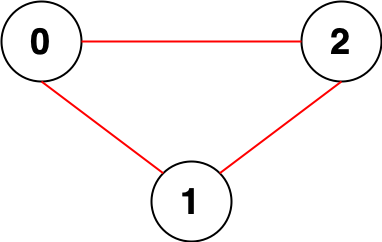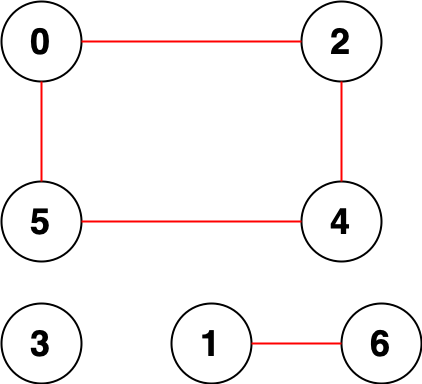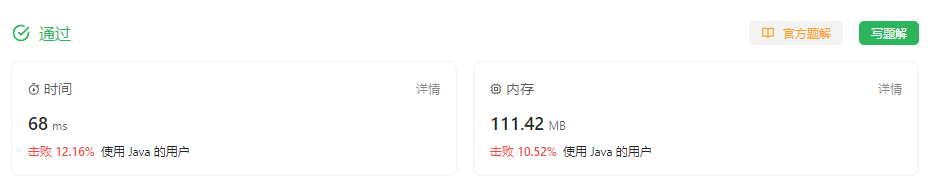#### 2316. 统计无向图中无法互相到达点对数

###### 来源: 每日一题 2023.10.21``````输入：n = 3, edges = [[0,1],[0,2],[1,2]]

````````````输入：n = 7, edges = [[0,2],[0,5],[2,4],[1,6],[5,4]]

[[0,1],[0,3],[0,6],[1,2],[1,3],[1,4],[1,5],[2,3],[2,6],[3,4],[3,5],[3,6],[4,6],[5,6]]

``````

• `1 <= n <= 10^5`
• `0 <= edges.length <= 2 * 10^5`
• `edges[i].length == 2`
• `0 <= ai, bi < n`
• `ai != bi`
• 不会有重复边。
``````class StockSpanner {
public long countPairs(int n, int[][] edges) {

}
}
``````

#### 分析与题解

• 邻接表 + 深度优先遍历

这个题目其实就是对无向图的邻接表的理解, 那么求两个点没有任何关联, 我们只要如果只要求出一条完整的边, 那么剩下所有的节点一定与这条完整的边不连接, 不连接的含义就是这条边上的节点与剩下的所有节点都是两两无法相互到达.

那么基于这样的理论, 我们假设这条边上的节点个数是 `m` 个, 那么对于这条边上的所有节点与剩下的节点两两无法相互到达的组合个数为 `m * (n - m)` .

另外, 假设 `节点1``节点2` 无法相互到达, 那么深度优先遍历 `节点1` 时,会计算一遍 `节点1``节点2`; 深度优先遍历 `节点1` 时, 同样会计算一遍 `节点1``节点2`. 所以最终结果我们需要除以2.

接下来, 我们看一下具体的解题过程.

首先, 我们先创建无向图的邻接表, 这里我使用的是HashMap来作为邻接表的存储空间.

``````// 创建邻接表
HashMap<Integer, ArrayList<Integer>> cache = new HashMap<>();
for(int i = 0; i < n; i++) {
cache.put(i, new ArrayList<>());
}
for(int[] item: edges) {
Integer first = item;
Integer second = item;
}
``````

然后通过深度优先遍历查找每一条边符合题目的个数.

``````// 深度优先遍历
// 当我们找到一个完整链路节点, 那么这些节点就不可能和剩下的节点有链接了
// 假设找到某条无线边的所有节点为m个, 总结点数为n个. 那么相互不能到达的两两节点数为 m * (n - m)
boolean[] visited = new boolean[n];
long result  = 0;
for(Integer key : cache.keySet()) {
if(!visited[key]) {
long count = dfs(key, cache, visited);
result += (n - count) * count;
}
}
``````

对于深度优先遍历, 我们就没有啥好说的, 我们只需要按照常规方式进行递归即可.

``````public int dfs(Integer key,  HashMap<Integer, ArrayList<Integer>> cache, boolean[] visited) {
if(visited[key]) {
return 0;
}
visited[key] = true;
int count = 1;
ArrayList<Integer> group = cache.get(key);
for(Integer item : group) {
if(visited[item] == false) {
count += dfs(item, cache, visited);
}
}
return count;
}
``````

然后最后的计算结果因为所有的个数都计算了两遍, 我们需要除以2来求出最终的结果.

``````return result/2;
``````

最后, 我们一起看一下整体的代码逻辑.

``````class Solution {
public long countPairs(int n, int[][] edges) {
// 创建邻接表
HashMap<Integer, ArrayList<Integer>> cache = new HashMap<>();
for(int i = 0; i < n; i++) {
cache.put(i, new ArrayList<>());
}
for(int[] item: edges) {
Integer first = item;
Integer second = item;
}
// 深度优先遍历
// 当我们找到一个完整链路节点, 那么这些节点就不可能和剩下的节点有链接了
// 假设找到某条无线边的所有节点为m个, 总结点数为n个. 那么相互不能到达的两两节点数为 m * (n - m)
boolean[] visited = new boolean[n];
long result  = 0;
for(Integer key : cache.keySet()) {
if(!visited[key]) {
long count = dfs(key, cache, visited);
result += (n - count) * count;
}
}
return result/2;
}

public int dfs(Integer key,  HashMap<Integer, ArrayList<Integer>> cache, boolean[] visited) {
if(visited[key]) {
return 0;
}
visited[key] = true;
int count = 1;
ArrayList<Integer> group = cache.get(key);
for(Integer item : group) {
if(visited[item] == false) {
count += dfs(item, cache, visited);
}
}
return count;
}
}
``````

复杂度分析:

• 时间复杂度: O(m + n), n 是总结点的个数, m是边数
• 空间复杂度: O(m + n)

结果如下所示.IT界无底坑洞栋主 欢迎加Q骚扰:676758285